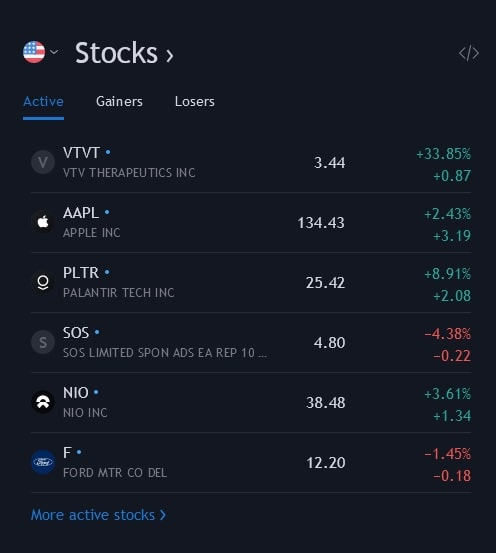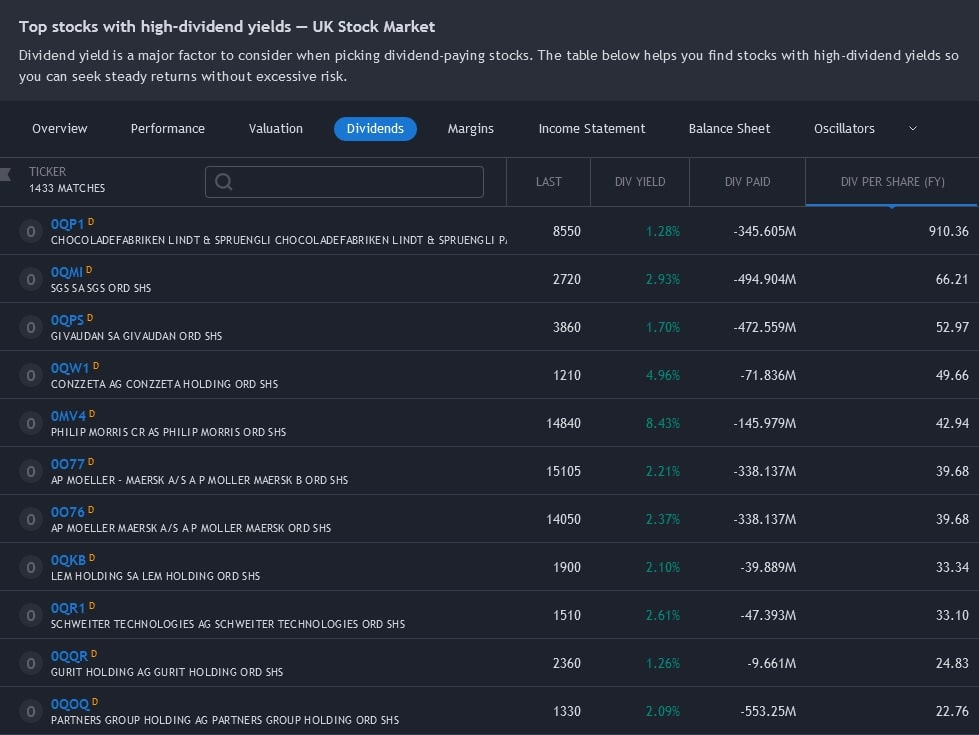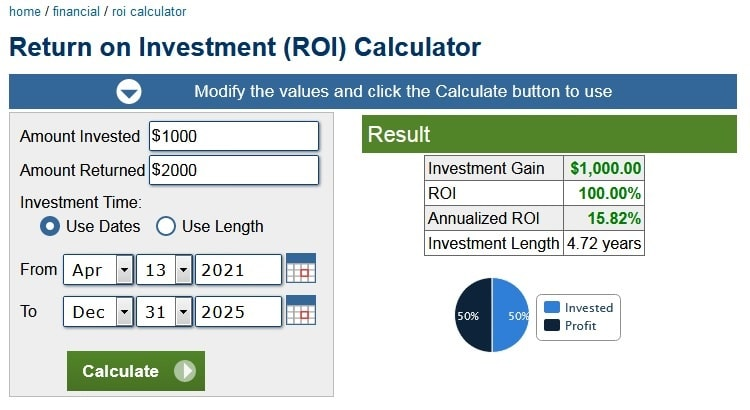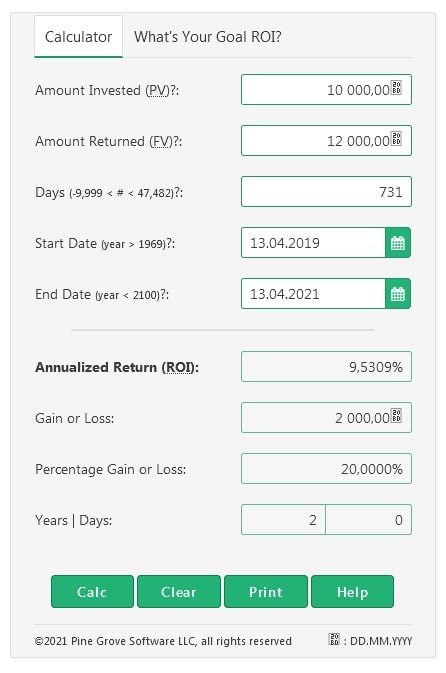# How to calculate ROI

16 Apr, 2021 Martin PetkovEvery investor wants to know how profitable their investments are. One of the most informative indicators that can answer this question is return on investment (ROI). In this article, we'll look at what it is, how to calculate the total and annualised return on investment for any asset and why it's worth doing so.

First, only invest in companies that have the potential to return the value of the entire fund. — Peter Thiel, entrepreneur and venture capitalist

## What is Return on Investment (ROI)?

Simply put, ROI is the ratio of money earned to money spent. It's an indicator that allows you to measure an investment's profitability and shows whether the investment has paid off. Because it's so simple to calculate and understand, this indicator is very popular among investors and business owners. ROI calculation applies to trading, investing and any business or purchase.

ROI is usually expressed as a percentage for ease of understanding and clarity. If the number is positive, the investment has made a profit. If it's negative, the investment turned is a loss.## How to calculate Return on Investment

There are different methods for calculating ROI. The simplest ROI calculation formula is:

ROI = Net Return on Investment / Cost of Investment * 100%

To determine the Net Return on Investment, you need to subtract Total Costs from Total Returns. If you are analysing investments in assets that bring dividends, such as shares, Total Returns is the sum of the dividends and the value of the shares at the time of sale. For investments in cryptocurrencies that do not bring dividends, you only consider the selling price. Total Cost is the asset's initial cost plus any other costs you incur in acquiring and owning the asset.

Other formulas used to calculate the ROI are derived from this basic formula.### Example of ROI calculation

Let's say you purchase 1,000 shares of company X at \$20 per share. A year later, you sell them at a higher price of \$24 per share. On top of that, you earn \$700 in dividends. You also spend a total of \$250 in commission when buying and selling shares.

In this case, the ROI calculation looks like this:

ROI = (((\$24 - \$20) * 1000 + \$700 - \$250) / (\$20 * 1000)) * 100 = 22.25%

We see that your investment turned out to be profitable, with an ROI of 22.25%.

ROI is calculated similarly for investments in other asset types. It's worth noting that the calculation will be a little more difficult for some assets, such as real estate, due to the larger number of expense items. When calculating ROI, it's important to consider all costs. Otherwise, the result will be inaccurate and probably misleading. On the other hand, when investing in cryptocurrencies, the calculation is more straightforward since investments in them don't bring dividends and the only type of income received by a crypto investor is capital gain.

### How to calculate annualised and monthly Return on Investment

The basic formula for calculating ROI has one significant limitation: it doesn't take into account the length of time the asset was owned. The Annualised ROI indicator allows us to correct this shortcoming. The formula for calculating it is:

Annualised ROI = ((1 + ROI / 100%) ^ 1/n − 1) * 100

Where n = number of days the asset was owned / 365

Monthly ROI is calculated in the same way. You only need to substitute the number of months instead of the number of years the asset was owned.

## Why you need to know ROI

Regular analysis of ROI is important for any investor. It helps determine the profitability of investments in different assets and how much sense it makes to continue holding these assets. ROI allows you to assume the expected profitability of investments, assess the investment portfolio's composition and compare different assets in terms of investment attractiveness. In addition, ROI can be calculated both for an asset as a whole and for each specific transaction with the asset.

ROI is an extremely popular metric because it's:

• Easy to calculate. The formula for calculating ROI is simple and doesn't require any special knowledge to use it.
• Easy to understand. This indicator's simplicity and clarity make it readily understandable to almost anyone and is used universally.

At the same time, ROI has several disadvantages to bear in mind when using it.

• Vulnerability to errors and manipulation. When calculating ROI, all costs may not be included. Moreover, this can be done by mistake or deliberately with the aim of misleading potential investors.
• Risk not factored in. One of the most important patterns inherent in investments is that the return on investment is directly proportional to risk. The higher the yield, the higher the risk, and vice versa. ROI doesn't measure risk in any way. Therefore, for any serious assessment of investments, additional research has to be done.
• Currency exchange rate changes not factored in. Over time, the price of the assets and the currency in which the assets are valued both change. ROI doesn't factor in the change in the exchange rate. Therefore, its results are inaccurate.
• Time not accounted for. As mentioned above, ROI doesn't consider the length of time an asset is held. Annualised ROI is used to correct this.

We can draw the following conclusion about ROI: it's an excellent option for quickly assessing an investment, which allows you to weed out unprofitable options immediately. However, you should never use ROI as the sole source of data for decision-making. This indicator reveals its potential only in conjunction with other indicators used in financial analysis.

## Return on Investment calculators

Because ROI is a popular indicator that may also be inconvenient to calculate manually, it's no surprise that many ROI calculators have popped up.

### Free online ROI calculators

There are now many online ROI calculators available for UK residents (and the rest of the world). They can be used both for financial assets and other property. Here are some of those ROI calculators.

1. ROI calculator on Calculator.net. A convenient calculator that computes both the Total and Annualised ROI, as well as Total Investment Gain.2. ROI calculator on Financial-calculators.com. Unlike the previous calculator, this one features a different interface and lets you print the result.### Calculating ROI in Excel

If you're an Excel user, you can easily use the formula and create your own Return on Investment calculator, taking into account all income and costs associated with your assets.

### Exchange BTC